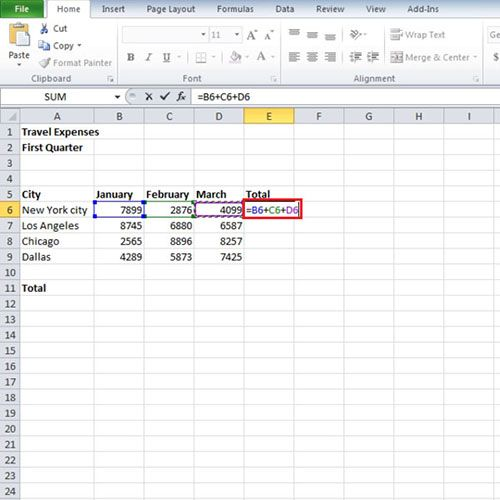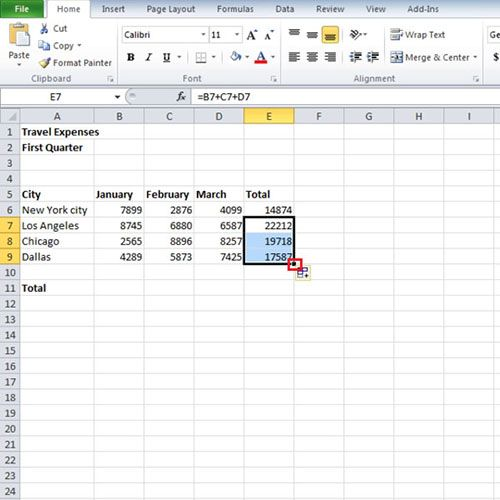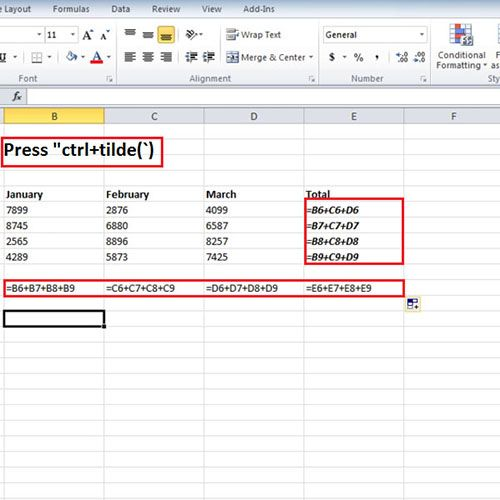## How to Add Numbers in Excel

Excel allows you to do various kinds of calculations. There are various formulas and functions that you can use for calculations. In order to add the number you can use the “SUM” function. In this tutorial we will not use the add formula in Excel, instead we will add the numbers manually and excel will recognize the pattern and do the calculations for rest of the cells itself.

Follow this step by step tutorial to learn how to add numbers in Excel.

Step # 1 – Add the values

In this tutorial we have a sheet in which we have few values which represent the expenses, city wise, which we incurred during the month January, February and March. In order to find the add numbers in Excel, under the heading of “Total” write “=B6 + C6 + D6”. After writing that, press the ‘Enter’ button and the result will be shown to you. The sum of all the three values will be calculated.Step # 2 – Use the Auto fill feature

This is how you can add formulas in Excel without using the SUM formula. When the sum of all the three values, which were from cell B6 till D6, is calculated, we can use the Auto-fill feature to take out the sum of the remaining cities. When you will click on cell in which you had entered the formula, a small plus sign will be shown to you at the bottom right corner of the cell. Press the mouse button and drag it or double click it to automatically calculate the rest of the sums.Step # 3 – Verify the formula

If you want to see the formulas that you have entered you can press “Ctrl + `”. This will show you how the final value, in this case the sum, is derived and which cells were used to calculate the sum. This feature is there so that you can verify or check whether the calculated value is taking the correct cells to do the calculations.